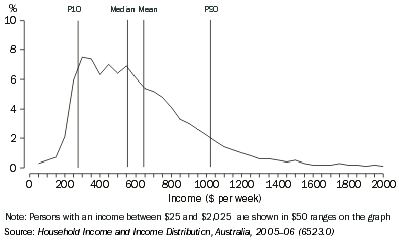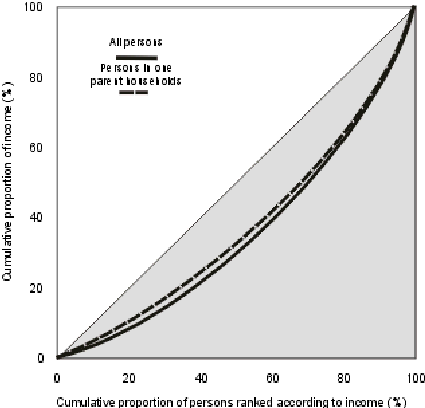6553.0 - Information Paper: Survey of Income and Housing, User Guide, Australia, 2005-06
Previous ISSUE Released at 11:30 AM (CANBERRA TIME) 13/08/2007
Page tools:Print AllRSSSearch this Product
Contents >> Part 1 Concepts and Definitions >> 1.6 Gini coefficient and other measures of income distribution

INTRODUCTION

There are many ways to illustrate aspects of the distribution of income and to measure the extent of income inequality. In the SIH, five main types of indicator are used - means and medians, frequency distributions, percentile ratios, income shares, and Gini coefficients. This section describes how these indicators are derived.

MEAN AND MEDIAN

Mean household income (average household income) and median household income (the midpoint when all persons or households are ranked in ascending order of household income) are simple indicators that can be used to show income differences between subgroups of the population.

The main income measure used in published SIH output is equivalised disposable household income, and the means and medians are person weighted. That is, they are calculated with respect to the relevant number of persons. This enables people in large households to have the same contribution to the mean/median as people living alone, and is possible because equivalised disposable household income is an indicator of the economic resources available to each individual in a household.

The method for calculating person weighted means and medians is described in section 2.7 'Calculation of population counts, means, medians and other estimates'.

In some tables describing households, the mean and median of gross household income are also shown. These measures are calculated with respect to the relevant number of households, not persons. They are sometimes known as household weighted measures.

FREQUENCY DISTRIBUTION

A frequency distribution illustrates the location and spread of income within a population. It groups the population into classes by size of household income and gives the number or proportion of people in each income range. A graph of the frequency distribution is a good way to portray the essence of the income distribution. Graph 1.6.1 below shows the proportion of people within \$50 household income ranges.

1.6.1 DISTRIBUTION OF EQUIVALISED DISPOSABLE HOUSEHOLD INCOME, 2005-06Frequency distributions can provide considerable detail about variations in the income of the population being described, but it is difficult to describe the differences between two frequency distributions. They are therefore often accompanied by other summary statistics, such as the mean and median. Taken together, the mean and median can provide an indication of the shape of the frequency distribution. As can be seen in the graph above, the distribution of income tends to be asymmetrical, with a small number of people having relatively high household incomes and a larger number of people having relatively lower household incomes. The greater the asymmetry, the greater will be the difference between the mean and the median.

QUANTILE MEASURES

When persons (or any other units) are ranked from the lowest to the highest on the basis of some characteristic such as their household income, they can then be divided into equally sized groups. The generic term for such groups is quantiles.

Quintiles, deciles and percentiles

When the population is divided into five equally sized groups, the quantiles are called quintiles. If there are 10 groups, they are deciles, and division into 100 groups gives percentiles. Thus the first quintile will comprise the first two deciles and the first 20 percentiles.

SIH publications frequently present data classified into income quintiles, supplemented by data relating to the 2nd and 3rd deciles combined. The latter is included to enable quintile style analysis to be carried out without undue impact from very low incomes which may not accurately reflect levels of economic wellbeing. (See section 1.5 'Low income households').

Equivalised disposable household income is the income measure used to define the quantiles shown in SIH publications, and the quantiles each comprise the same number of persons, that is, they are person weighted.

Upper values, medians and percentile ratios

In some analyses, the statistic of interest is the boundary between quantiles. This is usually expressed in terms of the upper value of a particular percentile. For example, the upper value of the first quintile is also the upper value of the 20th percentile and is described as P20. The upper value of the ninth decile is P90. The median of a whole population is P50, the median of the 3rd quintile is also P50, the median of the first quintile is P10, etc.

Percentile ratios

Percentile ratios summarise the relative distance between two points on the income distribution. To illustrate the full spread of the income distribution, the percentile ratio needs to refer to points near the extremes of the distribution, for example, the P90/P10 ratio. The P80/P20 ratio better illustrates the magnitude of the range within which the incomes of the majority of the population fall. The P80/P50 and P50/P20 ratios focus on comparing the ends of the income distribution with the midpoint (the median).

INCOME SHARES

Income shares can be calculated and compared for each income quintile (or any other subgrouping) of a population. The aggregate income of the units in each quintile is divided by the overall aggregate income of the entire population to derive income shares.

GINI COEFFICIENT

The Gini coefficient is a single statistic which summarises the distribution of income across the population.

The Gini coefficient can best be described by reference to the Lorenz curve. The Lorenz curve is a graph with the horizontal axis showing the cumulative proportion of the persons in the population ranked according to household income and with the vertical axis showing the corresponding cumulative proportion of equivalised disposable household income. The graph then shows the income share of any selected cumulative proportion of the population, as can be seen below in graph 1.6.2.

1.6.2 LORENZ CURVESIf income were distributed evenly across the whole population, the Lorenz curve would be the diagonal line through the origin of the graph. The Gini coefficient is defined as the ratio of the area between the actual Lorenz curve and the diagonal (or line of equality) and the total area under the diagonal. The Gini coefficient ranges between zero when all incomes are equal and one when one unit receives all the income, that is, the smaller the Gini coefficient the more even the distribution of income.

Normally the degree of inequality is greater for the whole population than for a subgroup within the population because subpopulations are usually more homogeneous than full populations. This is illustrated in the graph above, which shows two Lorenz curves from the 2005-06 SIH. The Lorenz curve for the whole population of the survey is further from the diagonal than the curve for persons living in one parent, one family households, with at least one dependent child. Correspondingly, the calculated Gini coefficient for all persons was 0.307 while the coefficient for the persons in the one parent households included here was 0.263.

The Gini coefficient is discussed in more detail, along with the Theil index and Atkinson index, in Appendix 3 'Gini coefficient and other single statistic summaries of income distribution'.

 Previous Page Next Page